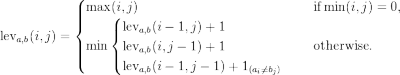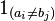Jans Blog

# Cleaning some data with Clojure 2

27.12.2014

Cleaning some data with Clojure 1” provides some context on this project. This post here focusses on the question

“Can we tell, if two strings are similar?”.

Levenshtein Distance: My first idea was to use the Levenshtein Distance. This metric counts the minimum number of operations needed to transform one string into the other when allowing insertion, deletion or substition of characters. Its definition is as follows:<figcaption class="wp-caption-text">Definition of Levenshtein Distance</figcaption></figure>denotes the indicator function, which is equal to 0 when the i-th character of a is equal to the j-th character of b. Otherwise it’s 1.

Transforming this into clojure-code is fairly simple, given the definitions. First, the indicator-function:

```(defn indicator
"0, if the i-th character of a and the j-th character of b
are the same. 1 otherwise."
[a b i j]
(if (= (get a (dec i)) (get b (dec j)))
0
1))
```

Next, a helper function which will be called recursively:

```(defn lev
"Helper function to compute the levenshtein distance"
[a b i j]
(if (zero? (min i j))
(max i j)
(min (inc (lev a b (dec i) j))
(inc (lev a b i (dec j)))
(+ (lev a b (dec i) (dec j)) (indicator a b i j)))))```

And finally the “public api”:

```(defn levenshtein
"Computes the Levenshtein distance between two strings a and b"
[a b]
(lev a b (count a) (count b)))
```

Unfortunately, this solution is not very performant . Also it blows the stack somewhere above 50 characters (tested for 140 characters). Nonetheless, this is enough for my use-case 😉

```=> (def alphabet "alphabetdefghijklmnopqrstuvwxyz")
#'/alphabet
=> (time (levenshtein (subs alphabet 0 10) (subs alphabet 0 10)))
"Elapsed time: 3855.856306 msecs"
0
=> (time (levenshtein (subs alphabet 0 11) (subs alphabet 0 11)))
"Elapsed time: 21493.424412 msecs"
0
```

We have this performance issue because most values are computed multiple times; some are computed extremely often. The solution in this case is a well-placed `memoize` to reuse the computed values: `(def lev (memoize lev))`. All in all we get a huge performance boost :

```=> (time (levenshtein (subs alphabet 0 11) (subs alphabet 0 11)))
"Elapsed time: 1.230304 msecs"
0
=> (time (levenshtein (subs alphabet 0 15) (subs alphabet 0 15)))
"Elapsed time: 1.280788 msecs"
0
=> (time (levenshtein alphabet alphabet))
"Elapsed time: 3.099704 msecs"
0
=> (time (levenshtein rnd_50_1 rnd_50_2))
"Elapsed time: 11.464978 msecs"
38
=> (time (levenshtein rnd_140_1 rnd_140_2))
StackOverflowError clojure.lang.AFn.applyToHelper (AFn.java:148)
```

Note, that “rnd_X_Y” are distinct pseudo-random strings that were “generated” by hitting the keyboard X times.

Extending this metric: This metric does not take into account that \”o is equivalent to ö. We can fix this by replacing these special values with more common ones before computing the distances:

```(defn cleanString
"A hacky cleaning function. Replaces unusual characters by more common ones."
[s]
(switch "\"A" "Ä"
(switch "\"O" "Ö"
(switch "\"U" "Ü"
(switch "\"a" "ä"
(switch "\"o" "ö"
(switch "\"u" "ü"
(switch "\\ss{}" "ß" s))))))))))))
```

And for a vector etc. of strings:

```(defn clean
"Cleans a vector of strings"
[strings]
(map cleanString strings))
```

From distance to similarity: Our next step is to convert distances between two strings into a “similarity value”. The Levenshtein distance of two strings is `(<= 0 (levenshtein a b) (max (count a) (count b)))`. Therefore we can convert a Levenshtein distance to a similarity metric as follows:

```(defn levensthein-similarity
"Computes a similarity measure based on Levenshtein distance. 0 ~ a and b completely different, 1 ~ a and b equal"
[a b]
(- 1 (/ (levensthein a b)
(max (count a) (count b)))))
```

TL;DR: Use an appropriate String Metric and convert it to a similarity metric. I tried out Levenshtein Distance which does not takes into account that \”o and ö are equivalent.

 “We should forget about small efficiencies, say about 97% of the time: premature optimization is the root of all evil.” (Knuth, Donald. Structured Programming with go to Statements, ACM Journal Computing Surveys, Vol 6, No. 4, Dec. 1974. p.268.); from clean-code-developer.de

 These numbers mean next to nothing: we don’t know yet if these improvements hold true for random strings. This will probably be on a later post.

Edit: Better code listings.